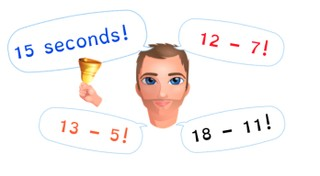Automaticity subtraction to 20 crossing tens

# Automaticity subtraction to 20 crossing tens

Learn to quickly and correctly subtract numbers to 20 crossing tens in your head

No account needed.8,000 schools use Gynzy92,000 teachers use Gynzy1,600,000 students use Gynzy

## General

Students learn to quickly and correctly subtract numbers to 20 crossing tens in their heads.

## Standards

CCSS.Math.Content.2.OA.B.2

## Learning objective

Students will be able to quickly and correctly subtract numbers to 20 crossing tens in their heads.

## Introduction

Practice two subtraction problems with the students by solving them using the visual support provided on the interactive whiteboard.

## Instruction

Explain to students what automaticity is, and what automaticity in subtraction problems means. Tell students that they are going to practice solving subtraction problems in their head within a given time. The goal is to solve the subtraction problem within 5, 4, and even 3 seconds. Start by discussing the three problems, the first in 5 seconds, then 4, and finally in 3 seconds. Then ask students to solve the problems within those times and discuss the answers. Tell students that have trouble solving within time that this is something that improves with practice. Then challenge the class to solve the set of three problems within 15 seconds and then four problems within 20 seconds.

Check that students are able to quickly and correctly solve subtraction problems to 10 by asking the following questions:
- Why is it useful to be able to quickly solve subtraction problems?
- How do you solve 12 minus 4 using the middle step to the multiple of ten?
- How can you make sure that you get better and faster at solving subtraction problems?

## Quiz

Students practice with sets of subtraction problems and are challenged to complete them in progressively less time.

## Closing

Have the class form a circle. Select one student who names a subtraction problem, closes their eyes, and spins in a circle. The student they point at when they stop spinning must give the answer to the problem in 5, 4, or 3 seconds. You can also remove the time limit, or have students name their subtraction problem after they spin, depending on the needs of your classroom.

## Teaching tips

Students who have difficulty solving the subtraction problems within 5 seconds can first be given the task of practicing subtraction problems to 20 without any time limits. Then you can start by having them solve a problem in 10 seconds, 8 seconds, 5 seconds, etc.

### The online teaching platform for interactive whiteboards and displays in schools

• Save time building lessons

• Manage the classroom more efficiently

• Increase student engagement